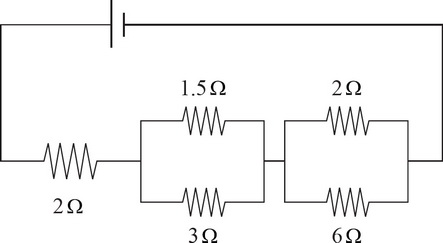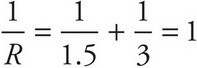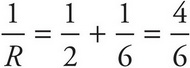# AP Physics 1 Question 304: Answer and Explanation

### Test Information

Question: 3042. What is the equivalent resistance of the electric circuit in the picture above?

• A. 0.46 Ω
• B. 1.2 Ω
• C. 4.5 Ω
• D. 14.5 Ω

Remember that parallel resistors require you to use the reciprocal sum, while series resistors simply add. For example, the first set of parallel resistors would be combined by doing, which means R = 1 Ω. For the second set of parallel resistors, you would get, which means R = 1.5 Ω. Adding those two values to the lone series resistor gives RTotal = 2 + 1 + 1.5 = 4.5 Ω.